# Next few terms in a sequence - consistent difference between differences

In order to work out the next few terms in a sequence when there is a consistent difference between differences follow the examples below: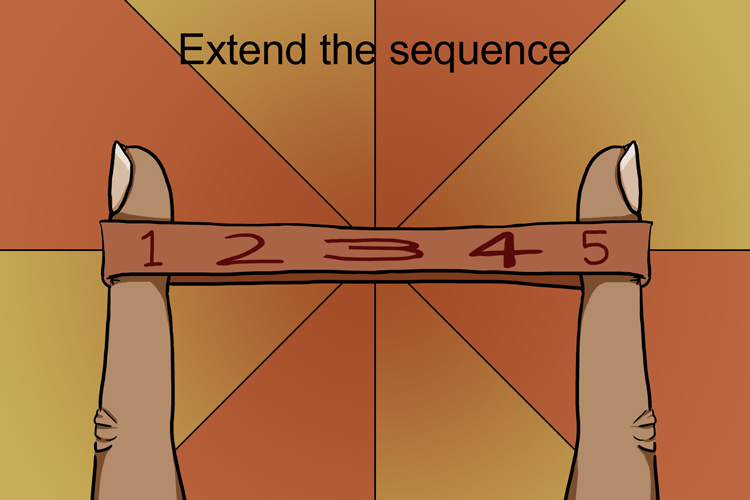Example 1

In the following sequence what are the next two sets of numbers?

2,   4,   8,   14,   22

First, see if there is a consistent difference between each number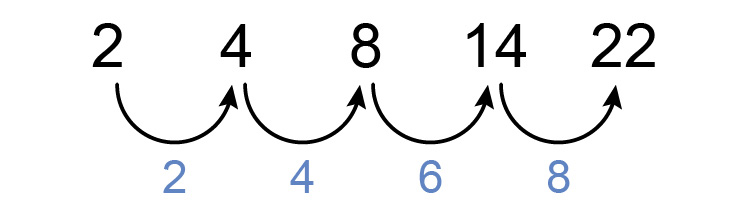There is not but now see if there is a consistent difference between differences.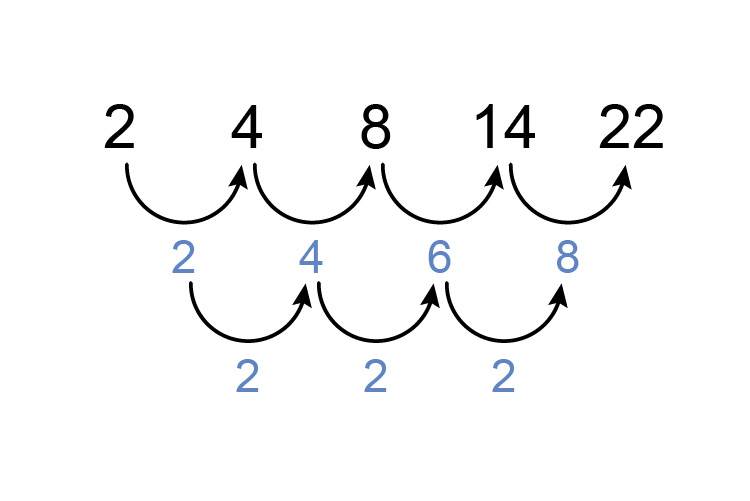There is! Great!

To find the next two sets of numbers extend the sequence in the same pattern in stages as follows:

First the bottom line.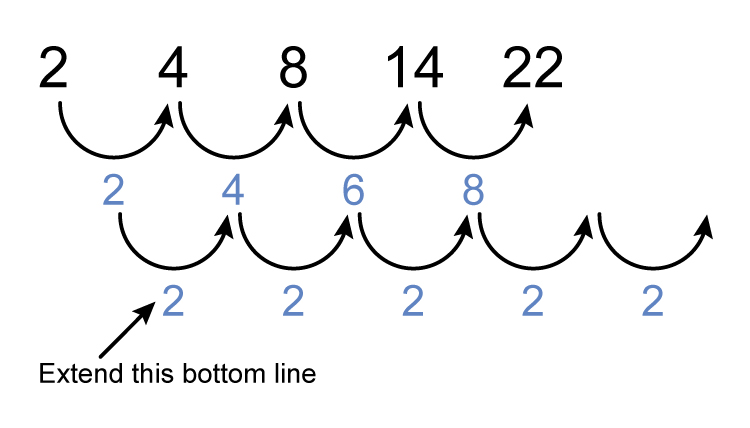Then the middle line.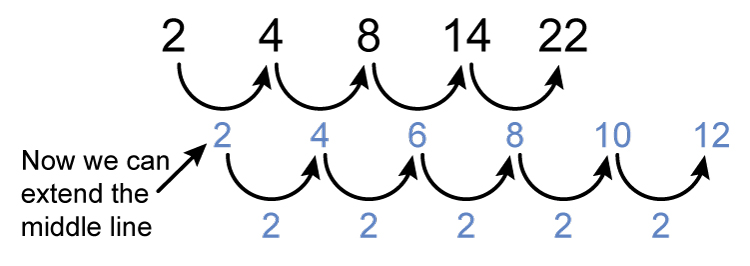Finally the sequence (the top line)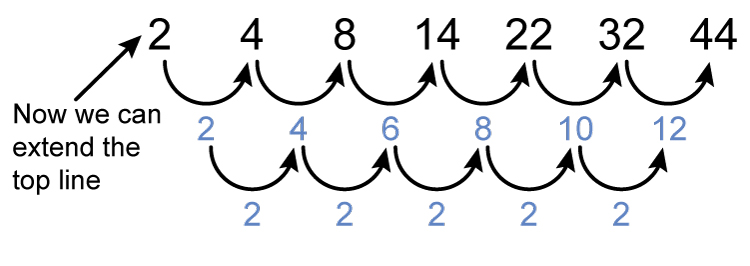Answer: The next two in the sequence are 32  and 44

Example 2

In the following sequence what are the next two sets of numbers?

6,   13,   26,   45,   70

First, see if there is a consistent difference between each number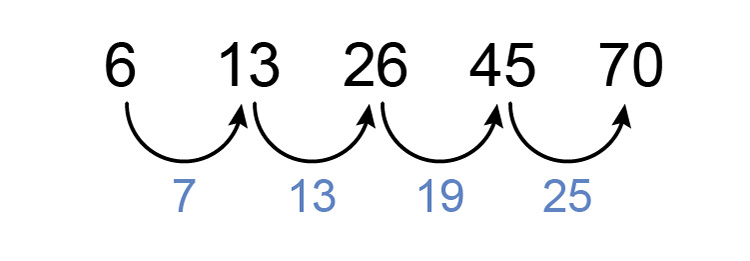There is not but now see if there is a consistent difference between differences.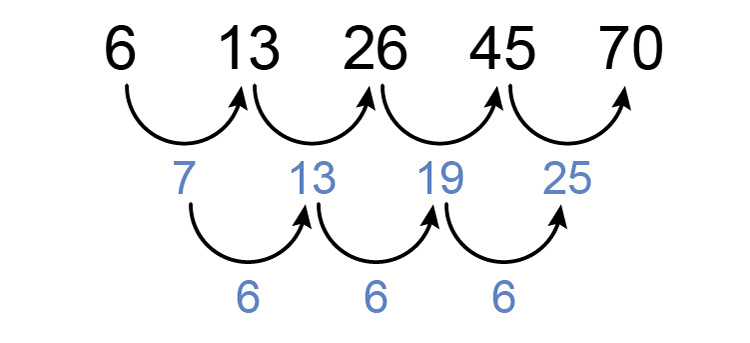There is! Great!

To find the next two sets of numbers extend the sequence in the same pattern in stages as follows:

First the bottom line.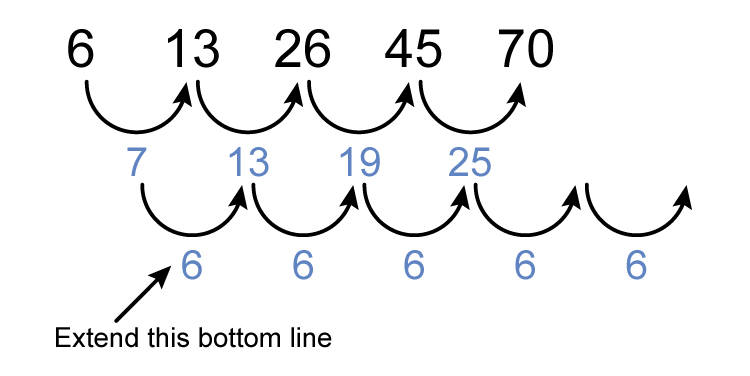Then the middle line.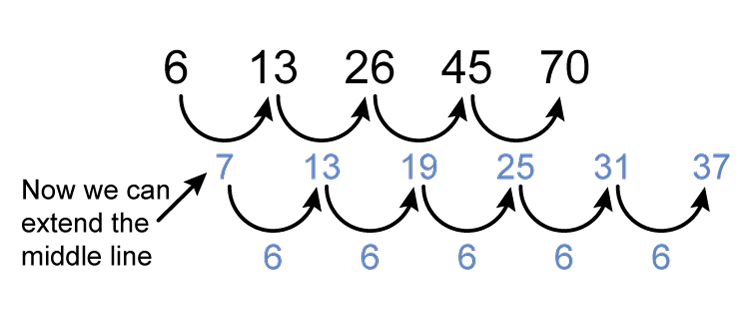Finally the sequence (the top line)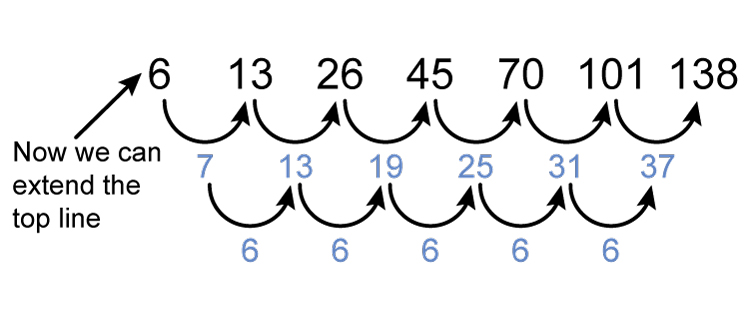Answer: The next two in the sequence are 101  and 138